Function Repository Resource:

# BouwkampDiagram

Convert Bouwkamp format into a diagram

Contributed by: Ed Pegg Jr
 ResourceFunction["BouwkampDiagram"][bouw] make a diagram out of Bouwkamp format bouw.

## Details

A Bouwkamp format can also be called a Bouwkamp code or Bouwkamp notation.
The Bouwkamp format is in the form of {order, y, x, s1, s2, …}, where order is the number of squares {s1, s2, …} in the diagram and y,x are the dimensions of the outer rectangle. The {s1, s2, …} are the side lengths of the squares comprising the diagram.
A sample Bouwkamp format is {12,16,16,3,3,5,5,6,4,6,7,3,1,5,4}. This means there are 12 squares filling out a 16×16 rectangle, with the first four squares (3,3,5,5) filling up the left side. All squares fill the leftmost lowest position until the rectangle is filled completely.

## Examples

### Basic Examples (2)

Turn a Bouwkamp format into a diagram:

 In:=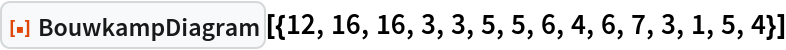Out=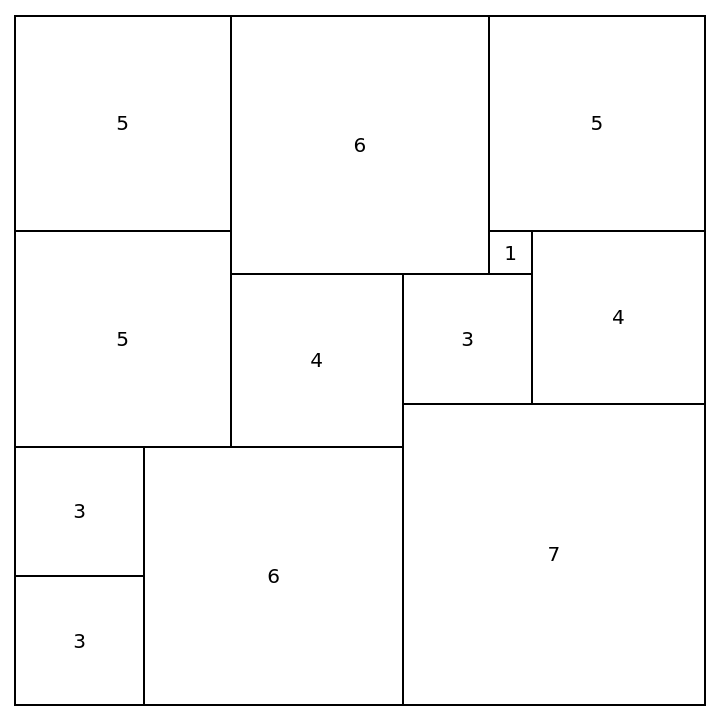Show the input form of the diagram:

 In:=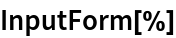Out=### Scope (2)

Show Moron's perfect rectangle (found in 1925):

 In:=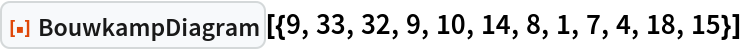Out=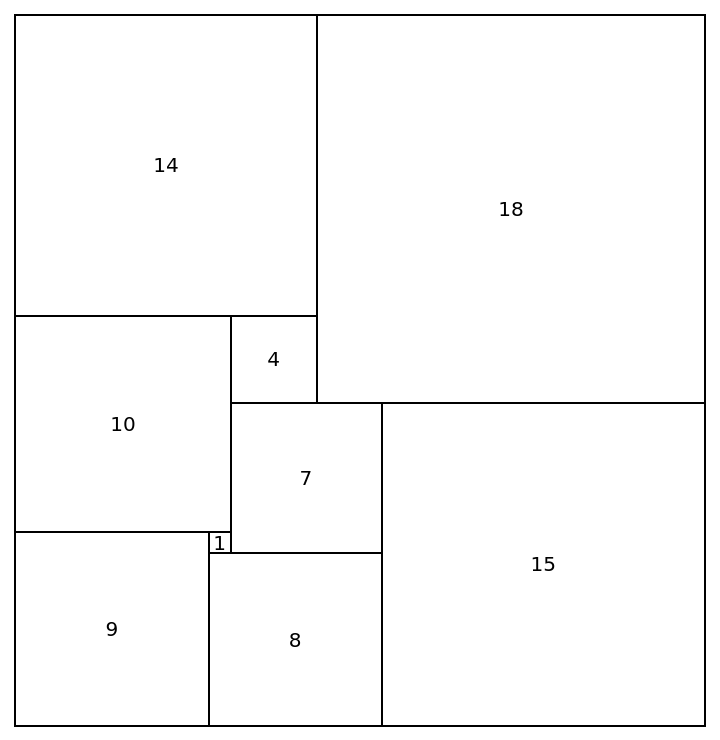Convert a more complicated Bouwkamp format:

 In:=Out=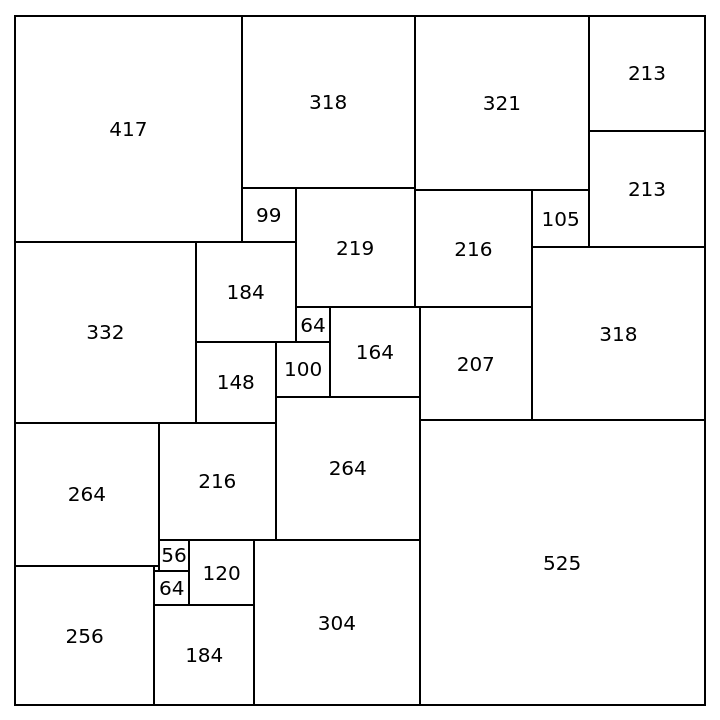### Possible Issues (1)

Invalid Bouwkamp formats will return a failure message:

 In:=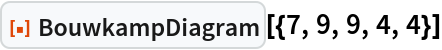Out=In:=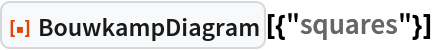Out=## Version History

• 1.0.0 – 25 January 2023